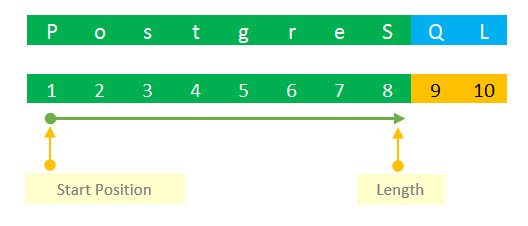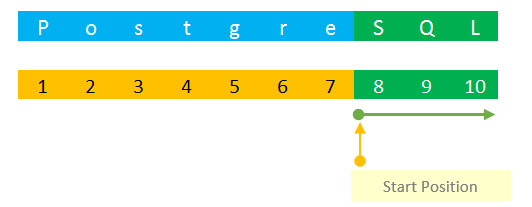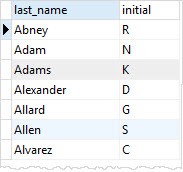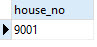# PostgreSQL Tutorial: Substring Function

August 2, 2023

Summary: in this tutorial, we will introduce you to PostgreSQL substring function that extracts a substring from a string.

## Introduction to PostgreSQL substring function

The substring function returns a part of string. The following illustrates the syntax of the `substring` function:

``````SUBSTRING ( string, start_position, length )
``````

Let’s examine each parameter in detail:

• string is a string whose data type is char, varchar, text, etc.
• start_position is an integer that specifies where you want to extract the substring. If `start_position` equals zero, the substring starts at the first character of the string. The `start_position` can be only positive. Though in other database systems such as MySQL the `substring` function can accept a negative `start_position`.
• length is a positive integer that determines the number of characters that you want to extract from the string beginning at `start_position`. If the sum of `start_position` and `length` is greater than the number of characters in the `string`, the substring function returns the whole string beginning at `start_position`. The length parameter is optional. If you omit the length parameter, the substring function returns the whole string started at `start_position`.

### PostgreSQL substring examples

See the following examples:

``````SELECT
SUBSTRING ('PostgreSQL', 1, 8); -- PostgreS
SELECT
SUBSTRING ('PostgreSQL', 8); -- SQL
``````

In the first statement, we extract a substring that has length of 8 and it is started at the first character of the `PostgreSQL` string. we get `PostgreS` as the result. See the following picture:In the second statement, we extract a substring started at position 8 and we omit the length parameter. The substring is a string beginning at 8, which is `SQL`.PostgreSQL provides another syntax of the substring function as follows:

``````substring(string from start_position for length);
``````

In this form, PostgreSQL puts three parameters into one. See the following example:

``````SELECT
SUBSTRING ('PostgreSQL' FROM 1 FOR 8); -- PostgreS
SELECT
SUBSTRING ('PostgreSQL' FROM 8); -- SQL
``````

The results are the same as the one in the first example.

In the following example, we query data from the customer table. We select `last_name` and `first_name` column. We get the initial name by extracting the first character of the `first_name` column.

``````SELECT
last_name,
SUBSTRING( first_name, 1, 1 ) AS initial
FROM
customer
ORDER BY
last_name;
``````## Extracting substring matching POSIX regular expression

In addition to the SQL-standard substring function, PostgreSQL allows you to use extract a substring that matches a POSIX regular expression. The following illustrates the syntax of the substring function with POSIX regular expression:

``````SUBSTRING(string FROM pattern)
``````

Or you can use the following syntax:

``````SUBSTRING(string, pattern);
``````

Note that if no match found, the substring function return a null value. If the pattern contains any parentheses, the substring function returns the text that matches the first parenthesized subexpression.

The following example extracts the house number (maximum 4 digits, from 0 to 9) from a string:

``````SELECT
SUBSTRING (
'The house no. is 9001',
'([0-9]{1,4})'
) as house_no
``````## Extracting substring matching a SQL regular expression

Besides POSIX regular expression pattern, you can use SQL regular expression pattern to extract a substring from a string using the following syntax:

``````SUBSTRING(string FROM pattern FOR escape-character)
``````

This form of `substring` function accepts three parameters:

• `string`: is a string that you want to extract the substring.
• `escape-character`: the escape character.
• `pattern`: is a regular expression wrapped inside escape characters followed by a double quote (`"`). For example, if the character `#` is the escape character, the pattern will be `#"pattern#"`. In addition, the `pattern` must match the entire `string`, otherwise, the substring function will fail and return a `NULL` value.

See the following examples:

``````SELECT SUBSTRING (
'PostgreSQL'
FROM
'%#"S_L#"%' FOR '#'
); -- SQL

SELECT SUBSTRING (
'foobar'
FROM
'#"S_Q#"%' FOR '#'
); -- NULLL
``````

PostgreSQL provides another function named `substr` that has the same functionality as the `substring` function.

## Summary

• Use the PostgreSQL substring functions to extract substring from a string.